# 13.6 Mehanisms with different joints

## 1.0 Introduction

#### 1.1 Definition

Mehanisms with different joints
Explain free grades of different mechanisms and than make program for mechanism with nine free grades, which will draw trace (curve) of the important point P on the end of the stick. Program will draw speed trace of the point P also. See VDI 2156.

#### 2.1 Introduction

Free grades are described in VDI 2156 standard. We need two objects in the space for crank movement. These two objects are treated, one with regard to another. Two objects in the space have 6 free grades (3 translations and 3 rotations).
The main parts of mehanism are: pedestal, joint, stick, crank, slide, ...

#### 2.3 Labeling

Every type of free grade is marked with a symbol:

• D-rotation (Drehung-from German)
• S-translation (Schiebung-from German)
• W-screwing (Windung,Scraubung-from German)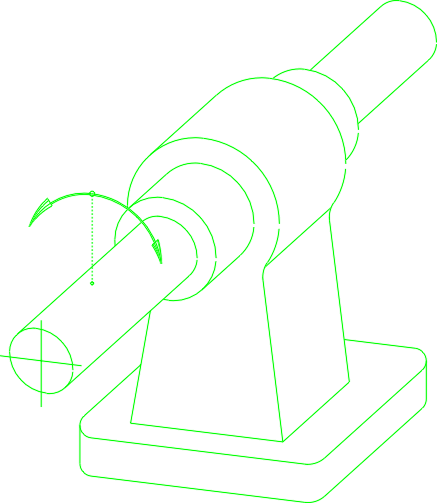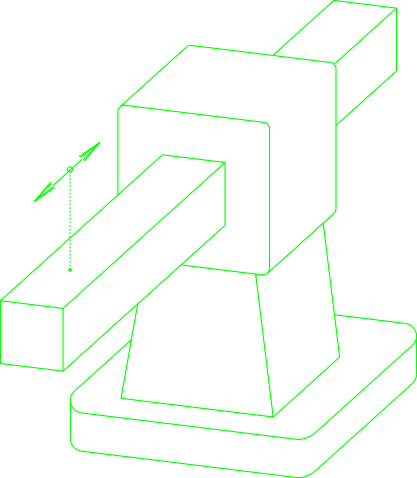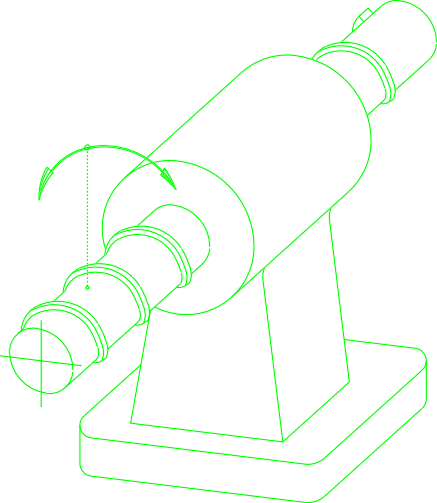In examples above we have only one free grade. With combination of letters and numbers we can describe more free grades together. For example:

• D3-mechanism with three rotations
• DS-mechanism with one rotation and one translation
• DS2-mechanism with rotation and two translations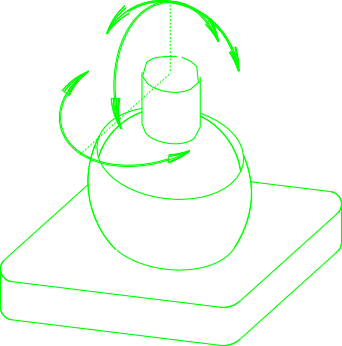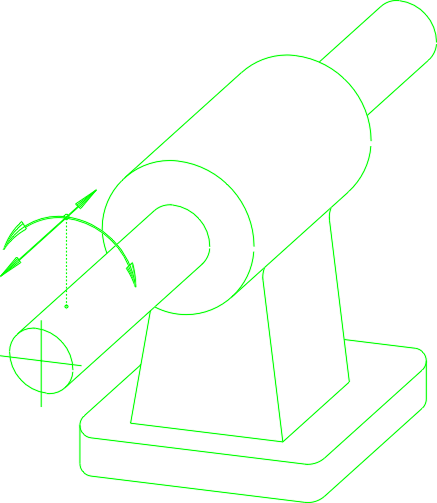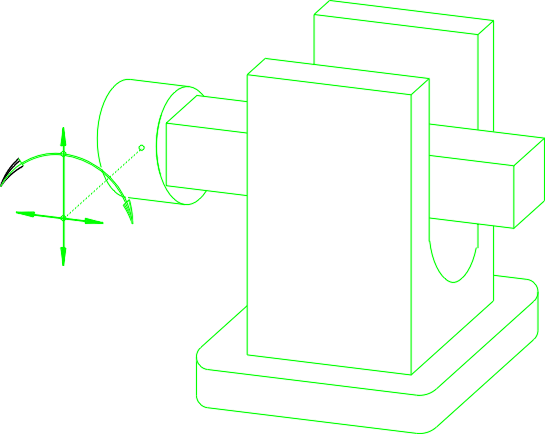## 3.0 Mechanism with 9 free grades

#### 3.1 Introduction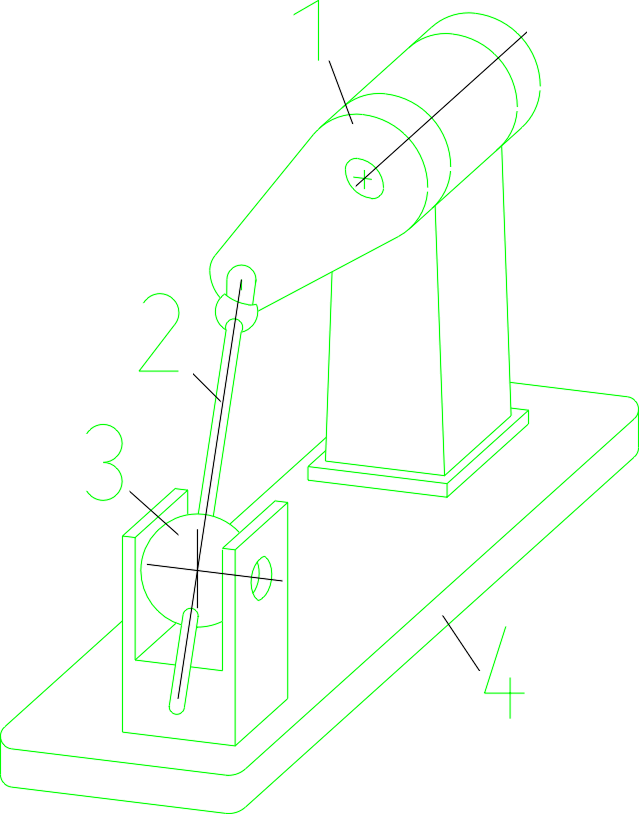D-D3=DS=D3
Mechanism is composed by many parts. From the picture above we can see, that the crank 1 is rotateing only around z axis, thatfor is marked with D. Stick 2 is fixed on crank 1 with joint which has three free grades (3 rotations), thatfor is marked with D3. Stick 2 has two free grades and is marked with DS. The sphere 3 has three rotations and marked by D3. If we put together this free grades, we get D-D3=DS=D3 (1+3+2+3=9) nine free grades mechanism.

#### 3.2 Maths basics

For understanding how program is working, you need to know how parameters (L, r, a, b, c, fi,...) efect on results. An important data is position of the sphere 3, which can be transformed by parameters a, b and c (see figure below)! The trace of important point P will be achived by transformation of points Pito points PiT. For this transformation we need an equation. Program wiil transform points Pi regardles to the position of the sphere 3 and other parameters L, r, fi,...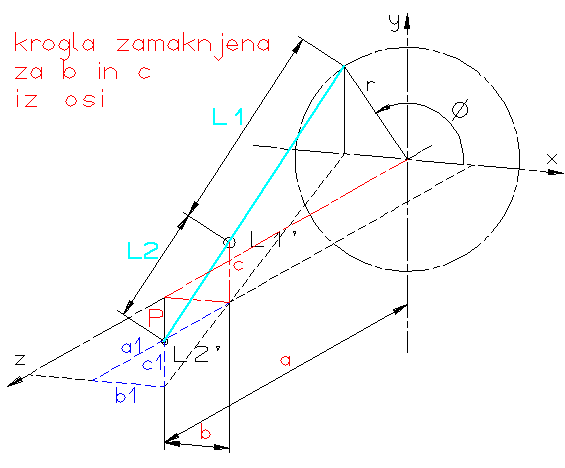If we put the sphere 3 in the same axis as rotateing crank 1, we get easy trace of point P (circle).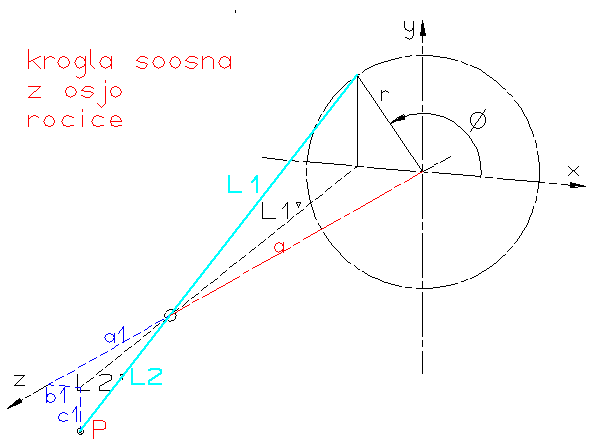To calculate coordinates of point P, are important resembling (similar) triangles. See figure below!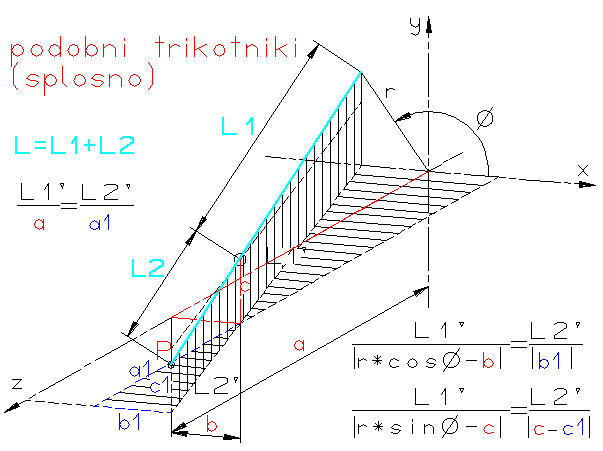## 4.0 Conclusion

#### 4.1 Conclusion

With this exercise I learned basics for programing with JavaScript and PHIGS.

#### 4.2 Literature

• Peter Hribar: Spoznajmo JavaScript
• Bojan Kraut : Strojniški priročnik